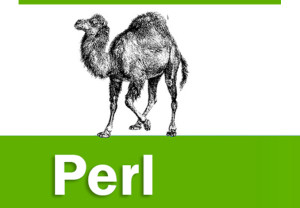31.How will you convert an array to string?
join() function joins the separate strings of LIST into a single string with fields separated by the value of EXPR, and returns the string.

join EXPR, LIST
Following is the example −

#!/usr/bin/perl

# define Strings
\$var_string = “Rain-Drops-On-Roses-And-Whiskers-On-Kittens”;
\$var_names = “Larry,David,Roger,Ken,Michael,Tom”;

# transform above strings into arrays.
@string = split(‘-‘, \$var_string);
@names = split(‘,’, \$var_names);

\$string1 = join( ‘-‘, @string );
\$string2 = join( ‘,’, @names );

print “\$string1\n”;
print “\$string2\n”;
print “\$string\n”; # This will print Roses
print “\$names\n”; # This will print Michael
This will produce the following result −

Rain-Drops-On-Roses-And-Whiskers-On-Kittens
Larry,David,Roger,Ken,Michael,Tom

32.How will you sort an array?
The sort() function sorts each element of an array according to the ASCII Numeric standards. This function has the following syntax −

sort [ SUBROUTINE ] LIST
This function sorts the LIST and returns the sorted array value. If SUBROUTINE is specified then specified logic inside the SUBTROUTINE is applied while sorting the elements.

#!/usr/bin/perl

# define an array
@foods = qw(pizza steak chicken burgers);
print “Before: @foods\n”;

# sort this array
@foods = sort(@foods);
print “After: @foods\n”;
This will produce the following result −

Before: pizza steak chicken burgers
After: burgers chicken pizza steak

33.What is the purpose of \$[ variable?
This special variable is a scalar containing the first index of all arrays. Because Perl arrays have zero-based indexing, \$[ will almost always be 0. But if you set \$[ to 1 then all your arrays will use on-based indexing. It is recommended not to use any other indexing other than zero. However, let’s take one example to show the usage of \$[ variable −

#!/usr/bin/perl

# define an array
@foods = qw(pizza steak chicken burgers);
print “Foods: @foods\n”;

# Let’s reset first index of all the arrays.
\$[ = 1;

print “Food at \@foods: \$foods\n”;
print “Food at \@foods: \$foods\n”;
This will produce the following result −

Foods: pizza steak chicken burgers
Food at @foods: pizza
Food at @foods: steak

34.How will you merge two arrays?
Because an array is just a comma-separated sequence of values, you can combine them together as shown below.

#!/usr/bin/perl

@numbers = (1,3,(4,5,6));

print “numbers = @numbers\n”;
This will produce the following result −

numbers = 1 3 4 5 6

35.How will you create Hashes in perl?
Hashes are created in one of the two following ways. In the first method, you assign a value to a named key on a one-by-one basis −

\$data{‘John Paul’} = 45;
\$data{‘Lisa’} = 30;
\$data{‘Kumar’} = 40;
In the second case, you use a list, which is converted by taking individual pairs from the list: the first element of the pair is used as the key, and the second, as the value. For example −

%data = (‘John Paul’, 45, ‘Lisa’, 30, ‘Kumar’, 40)

36.How will you get element from Hashes in perl?
When accessing individual elements from a hash, you must prefix the variable with a dollar sign (\$) and then append the element key within curly brackets after the name of the variable. For example −

#!/usr/bin/perl

%data = (‘John Paul’ => 45, ‘Lisa’ => 30, ‘Kumar’ => 40);

print “\$data{‘John Paul’}\n”;
print “\$data{‘Lisa’}\n”;
print “\$data{‘Kumar’}\n”;
This will produce the following result −

45
30
40

37.How will you get all keys from Hashes in perl?
You can get a list of all of the keys from a hash by using keys function, which has the following syntax −

keys %HASH
This function returns an array of all the keys of the named hash. Following is the example −

#!/usr/bin/perl

%data = (‘John Paul’ => 45, ‘Lisa’ => 30, ‘Kumar’ => 40);

@names = keys %data;

print “\$names\n”;
print “\$names\n”;
print “\$names\n”;
This will produce the following result −

Lisa
John Paul
Kumar

38.How will you get all values from Hashes in perl?
You can get a list of all of the values from a hash by using values function, which has the following syntax −

values %HASH
This function returns an array of all the values of the named hash. Following is the example −

#!/usr/bin/perl

%data = (‘John Paul’ => 45, ‘Lisa’ => 30, ‘Kumar’ => 40);

@ages = values %data;

print “\$ages\n”;
print “\$ages\n”;
print “\$ages\n”;
This will produce the following result −

30
45
40

39.How will you check if key exists in a hash or not?
Using the exists function, which returns true if the named key exists, irrespective of what its value might be −

#!/usr/bin/perl

%data = (‘John Paul’ => 45, ‘Lisa’ => 30, ‘Kumar’ => 40);

if( exists(\$data{‘Lisa’} ) ){
print “Lisa is \$data{‘Lisa’} years old\n”;
}
else{
print “I don’t know age of Lisa\n”;
}
Here we have introduced the IF…ELSE statement, which we will study in a separate chapter. For now you just assume that if( condition ) part will be executed only when the given condition is true otherwise else part will be executed. So when we execute the above program, it produces the following result because here the given condition exists(\$data{‘Lisa’} returns true −

Lisa is 30 years old

40.How will you get the size of hash?
You can get the size – that is, the number of elements from a hash by using the scalar context on either keys or values. Simply saying first you have to get an array of either the keys or values and then you can get the size of array as follows −

#!/usr/bin/perl

%data = (‘John Paul’ => 45, ‘Lisa’ => 30, ‘Kumar’ => 40);

@keys = keys %data;
\$size = @keys;
print “1 – Hash size: is \$size\n”;

@values = values %data;
\$size = @values;
print “2 – Hash size: is \$size\n”;
This will produce the following result −

1 – Hash size: is 3
2 – Hash size: is 3